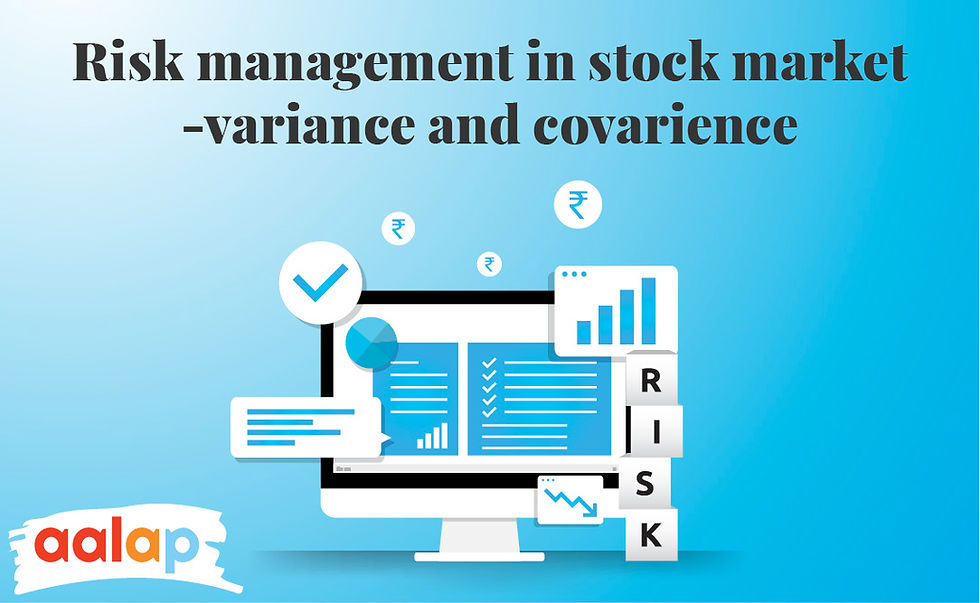top of page

# Risk management in stock market variance and covarianceRisk management in stock market is inevitable if you trade. Every rupee of profit gained by a trader must always be borne by a loss of rupee. As an extension, if one group of traders continuously makes money, another group must consistently lose money. Typically, the number of traders who continuously make money is tiny compared to the group of traders who constantly lose money.

The common definition of risk in the stock market is the "chance of losing money."

Risk management in stock market is important when you trade in the markets and open yourself to risk, which indicates you might (and probably will) lose money. That is why you should understand the risk management system in stock market.

For example, whether you like it or not, when you buy a company's shares, you expose yourself to risk. Furthermore, the risk may be classified at a high level into two types: systemic risk and Unsystematic risk. When you hold a stock, you are inherently exposed to both types of risks.

All of the below are examples of risk. In reality, there might be some additional causes, and the list could go on. However, all of these risks have in common that they are all company-specific. Let us consider some of the points of risk management in stock market,

• Incompetence in management

• Eating margins in competition

## Risk Management System in Stock market

It is the process of discovering, analyzing, and controlling risks to an organization's capital and profitability is known as "risk management". These risks arise from various causes, including financial uncertainty, legal liability, technological challenges, strategic management failures, accidents, and natural disasters.

A strong Risk Management System in stock market assists a business in considering all of the risks it confronts. Risk management also investigates the link between risks and their potential to have to influence an organization's strategic goals.

It is a management system that should be integrated into the organization's overall strategy. To connect them, risk management professionals must first identify the organization's risk appetite or the amount it needs to achieve its goals.

The point or steps considered in the risk management in the stock market are,

• Identification and Evaluation.

• Examine each one's possibility and impact.

• Risks should be prioritized depending on company objectives.

• The circumstances must be treated (or responded to).

• Keep an eye on the findings and make adjustments as needed.

When assessing risks, you should know that it has an effect.

## Risk Management Variance

Variance is a popular risk assessment measure. Investors usually calculate the variance of an expected return to estimate the relative risk of various investment situations. Project managers calculate variance to evaluate whether a project is over budget or behind schedule.

You can find out Risk Management Variance by adopting several methods.

### 1. Historical Data:

• Divide the total of the data set by the number of data points to get the average of the data set. There are three data points in this example: n1, n2, and n3:

difference 1 = (n1 - average) diff 2 = (n2 - average) diff 3 = (n3 - average)

• Each difference is squared, and the sum of the squared differences is:

[(n1 - average) ^2] + [(n2 - average)^2] + [(n3 - average)^2]

• Subtract the total of the squared differences from the number of data points in the set:

[(n1 - average) ^2] + [(n2 - average )^2] + [(n3 - average )^2] / (3-1)

### 2. Monte Carlo Method:

When assessing the risk variance of a proposed investment scenario, select a distribution that corresponds to the observed performance of previous investments.

Choose a statistical distribution to estimate the factors that impact your data collection.

Depending upon the statistical distribution that you selected, you will need to generate a random number from 1,000 to 10,000 in this method.

### 3. Variance Covariance Method:

• You need to multiply the standard deviation by 1.65 to calculate the risk that happens 5% of the time.

• You need to multiply the standard deviation by 1.65 to calculate the risk that happens 5% of the time.

• Multiply the standard deviation by 2.33 to calculate the risk that happens 1% of the time.

## Covariance vs Variance Formula

Variance and covariance are two mathematical concepts often used in statistics and probability theory. The spread of a data set about its mean value is referred to as variance, whereas covariance measures the directional connection between two random variables.

Risk Management Variance:

• S2 = Simple variance

• Σ = Sum

• Χ = Each Value

• x̄ = Simple mean

• n = sample’s value

The covariance vs variance formula information is given below,

• σ2 = Σ (Χ - μ)2 / N

• σ2 = Variance of Population

• Σ = Sum

• Χ = Each Value

• N = Population’s value number

Covariance,

• Cov (X ,Y) = E [(X−EX) (Y−EY)] = E [XY]−(EX) (EY)

## Summary

In addition to their broad application in statistics, both the phrases, covariance, and variance, have special connotations for investors related to stock market metrics and asset allocation. So the formula about the covariance vs variance formula is for the information you should know. You should know how the risk management variance and covariance generate their value.